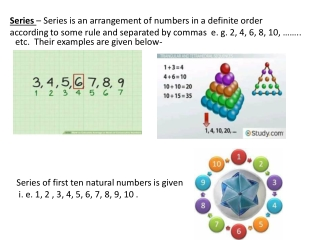DownloadDownload PresentationSeries.

# Series.

Download Presentation## Series.

- - - - - - - - - - - - - - - - - - - - - - - - - - - E N D - - - - - - - - - - - - - - - - - - - - - - - - - - -
##### Presentation Transcript

1. Series – Series is an arrangement of numbers in a definite order according to some rule and separated by commas e. g. 2, 4, 6, 8, 10, …….. etc. Their examples are given below- Series of first ten natural numbers is given i. e. 1, 2 , 3, 4, 5, 6, 7, 8, 9, 10 .

2. A. P. Series A series a1, a2, a3, ……….. anis called an A. P. series or an arithmetic progression if their exists a constant number ‘ d’ such that a2= a1+ d a3= a2+ d a4= a3+ d ……………… an= an-1+ d In this A. P. series common difference is 1.

3. nth Term of an A. P. If ‘ a’ is the first term and ‘d’ is the common difference of an A. P. then its nth term is given by an= a + (n-1 )d we can find the 20thterm of A. P. which is given below

4. Sum of n terms of A. P. The sum of n terms of an A. P. with first term ‘ a’ and the common difference ‘ d’ is Sn= n/2 ( 2a + (n-1 )d ) where n is the number of terms in an A. P. The formula to calculate sum of n terms of A. P. is given -